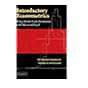Normal view MARC view ISBD view

# Econometric analysis / William H. Greene.

Material type:TextPublisher: Upper Saddle River, N.J. : Prentice Hall, c2000Edition: 4th ed.Description: xxv, 1004 p. : ill. ; 25 cm. +.ISBN: 0130132977; 9780130132970.DDC classification: 330.015195 Online resources: WorldCat details
Contents:
Table of contents Introduction -- Matrix algebra -- Probability and distribution theory -- Statistical inference -- Computation and optimization -- Classical multiple linear regression model: specification and estimation -- Inference and prediction -- Functional form, nonlinearity, and specification -- Large-sample results and alternative estimators for the classical regression model -- Nonlinear regression models -- Nonspherical disturbances, generalized regression, and GMM estimation -- Heteroscedasticity -- Autocorrelated disturbances -- Models for panel data -- Systems of regression equations -- Simultaneous-equations models -- Regressions with lagged-vriables -- Time-series models -- Models with discrete dependent variables -- Limited dependent variable and duration models.
Tags from this library: No tags from this library for this title.Average rating: 0.0 (0 votes)
Item type Current location Collection Call number Copy number Status Date due Barcode Item holdsCDs & DVDs
Audio Visual
Non-fiction 330.015195 GRE 2000 (Browse shelf) C-1 Available CD-1495Text
Reserve Section
Non-fiction 330.015195 GRE 2000 (Browse shelf) C-1 Not For Loan 26962
Total holds: 0
##### Browsing EWU Library Shelves , Shelving location: Audio Visual Close shelf browser321.3821 OLI 2005 Implementing 802.11, 802.16, and 802.20 wireless networks : 327.5405491 IND 2012 India-Pakistan relations, 1947-2007 : 330.01518282 BAI 2006 Introductory econometrics : 330.015195 GRE 2000 Econometric analysis / 330.015195 GUB 2003 Basic econometrics / 330.015195 GUB 2003 Basic econometrics / 330.015195 GUB 2003 Basic econometrics /

Includes bibliographical references (p. 963-988) and indexes.

Matrix algebra --
Probability and distribution theory --
Statistical inference --
Computation and optimization --
Classical multiple linear regression model: specification and estimation --
Inference and prediction --
Functional form, nonlinearity, and specification --
Large-sample results and alternative estimators for the classical regression model --
Nonlinear regression models --
Nonspherical disturbances, generalized regression, and GMM estimation --
Heteroscedasticity --
Autocorrelated disturbances --
Models for panel data --
Systems of regression equations --
Simultaneous-equations models --
Regressions with lagged-vriables --
Time-series models --
Models with discrete dependent variables --
Limited dependent variable and duration models.

Economics

There are no comments for this item.